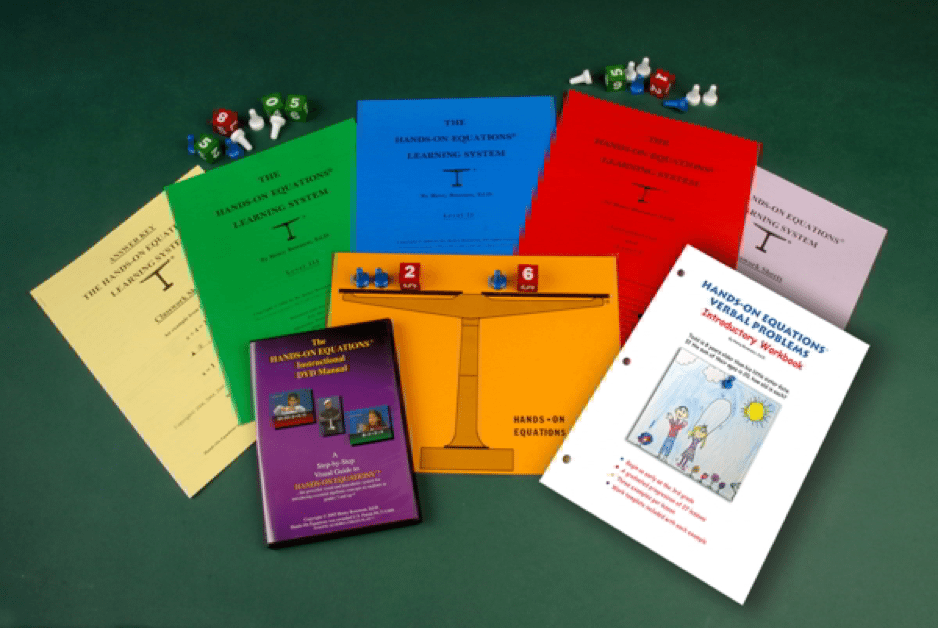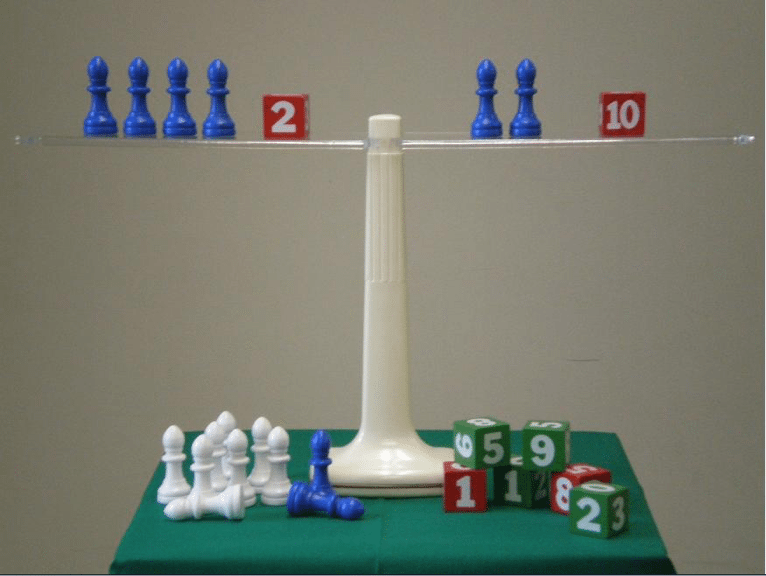# Hands-On Equations Math Curriculum ReviewIs it over the top to say that Hands On Equations Math Curriculum is a miracle product?  Because I really think it is. Why do I think this?  Because it teaches kids in grades 3 and up to solve algebraic equations like this one:  2X – 3(-X) + (-4) = 2(-X) +3.  And not only can kids solve this equation, they have fun doing so!  Don’t you wish you had this program when you were younger?  Would it have changed your life?  I know it would have changed mine. Read on to learn more about the Hands On Equations Math Curriculum Review we did!This Hands On Equations Math Curriculum Deluxe Home Set is everything you need to get your child started with algebraic equations and word problems. This set includes the Hands On Equations Learning System with reproduction rights for immediate family members, the Instructional Video Manual in DVD format, and the Hands On Equations Introductory Verbal Problems Workbook.

#### HANDS ON EQUATIONS MATH CURRICULUM DELUXE HOME SET – Price: \$54.95

Available from Borenson and Associates, Inc. at www.borenson.com

Let me explain a bit about the program:

The Hands-On Equations Learning System provides students in 3rd-8th grade (and beyond) with a concrete, visual and kinesthetic approach to working with algebraic linear equations. It enables students to grasp algebraic concepts concretely, pictorially and then abstractly.

That’s because students are taught how to transform an abstract equation into its physical counterpart, and then solve the equation through the use of “legal moves” (the physical counterparts of the mathematical principles involved). The program is game-like, but without points, winning or racing.

Kids learn that what they do to one side of the scale (you get a laminated scale sheet in the deluxe package), they have to do to the other, so it remains balanced.  Plus, kids learn the following mathematical concepts: the concept of an unknown, the concept of combining like terms, the meaning of the symbols used in an algebraic equation, how to check an answer, essential properties of equations such as the addition and subtraction properties of equality, essential concepts related to zero, an understanding of the relationship between an unknown quantity and its opposite, the addition and subtraction of positive and negative numbers, and more.

Wow!

The Hands On Equations Math Curriculum consists of  3 levels and 26 lessons (#26 is optional).  The lessons are presented via a DVD. Lesson one teaches kids to use a symbol to represent an unknown.  By lesson 26, students are solving equations like the bolded one above.  Specifically:

• Level 1 (7 lessons) – kids reinforce their computational skills, develop problem-solving skills, and gain confidence in algebraic reasoning. They solve equations such a 2(X+4) +X = X +16.
• Level 2 (lessons 8-16) – students gain familiarity with positive and negative numbers and solve equations such as 2X – 3(-X) = 20 + X.
• Level 3 (Lessons 17-25) – students progress to equations such as 2X – 2(-X +4) = X + (-2).

The research regarding this program is phenomenal. Really, click here and have a read.  4th graders do as well as 6th and 8th graders – which is pretty amazing.

Regarding the Verbal Problems  Introductory Workbook – the book has a graduated progression of 27 lessons (3 examples per lesson), plus two bonus lessons, and a work template is included with each example.  Answers are in the back of the book.  Kids should complete Level 1 of Hands-On Equations before beginning this book.

Here are examples of problems in the book –

If 3 is added to twice a number, the result is 17. Find the number (Level 1)

Four times a number, diminished by 6, yields the same result as twice the same number, increased by 4.  Find the number (Level 3)

Kids answer the question with a full sentence (including verb and subject).

With the Hands On Equations Math Curriculum, students learn to:

• Analyze verbal problems and determine what strategy is best for representing the problem using concrete models or pictorial symbols
• Use mathematical models to represent and solve application problems involving quantitative relationships with whole numbers
• Use multiple strategies to solve real-world application problems. Strategies include using a 5-step problem solving process, breaking a problem into parts and determining whether a problem has too much info or not enough
• Use substitution and mental math to check solutions to algebraic equations in one variable
• And MORE

What do I like about the Hands On Equations Math Curriculum Deluxe Home Set?

• EVERYTHING!
• Kids get a strong foundation in algebraic concepts – this sets them up for success when they take future math/algebra classes.
• This strong foundations gives kids confidence in their math abilities. That’s HUGE!
• Because it’s game-like – it’s not intimidating. Wow – all those Xs and Ys, and abstract theories are not intimidating!  Again, that’s HUGE!
• On the lesson videos, Dr. Borenson explains/teaches, but so do kids. Molly and Eric also explain/teach.  Molly is 11, and Eric is 8.  This sends a clear message to your kids – if they can do it, so can you!
• Each lesson teaches only one new concept. So, there’s no info overload.
• Good for all learning styles.
• New work and previous work are reviewed on lesson worksheets – I like the review aspect.
• Students are taught that they can solve equations in multiple ways.
• You get a lot with the deluxe edition (I recommend going deluxe) – a DVD, worksheets, answer sheets, Level 1-3 pamphlets for the parent, a laminated scale, the verbal problems workbook, etc.  After watching the videos, I do want the stationery physical scale (see below). I don’t care if it’s a bit pricey – I want it.  My kids want it.  And since the program is so inexpensive, I think I can splurge on the scale.
• With the Verbal Problems Introductory Workbook, children use full sentences when answering the question (must have a subject and a verb) – so they’re doing English and math at the same time.  I’m a fan of multi-tasking.
• Kids learn how to do the math – they learn the theories – but don’t have to memorize the names of the theories (for instance, the additive identity property). I know they need this info at some point due to testing, but they don’t need to be worried about it now.  What’s important is that they understand the theory – not the name of the theory.
• It’s a nice quality workbook – wipe-off cover, heavy paper. In fact, all the physical products are nice .
• Need more resources? There are FREE videos on their website and on YouTube.
• This is a physical product and there are digital downloads as well – I like having the option and convenience of grabbing worksheets digitally if I need them.
• This is a re-usable product – ALL of your children can use it. What a deal.  You might have to buy additional worksheets if you don’t make copies – I made copies from the originals.
• It’s so inexpensive for all that you get – and again, one set can be used for ALL of your children. So it’s really a deal.Hands On Equations Math Curriculum – Teacher’s Demonstration Scale and Game Pieces. Price: \$76.00

This demonstration scale is a stationary scale with no moving parts. It is used to illustrate equations. Comes with game pieces.Here’s the pic of all the resources that were a part of this Hands-On Equations product review.

In conclusion, the Hands On Equations Math Curriculum is an incredible product.

On a personal level – I didn’t do well in Math until I went to college.  Then, after a professor took me aside and gave me a bit of confidence, I excelled.  In high school though, it was a struggle.  It wouldn’t have been, had my teacher been using this program.  Heck, I could have conquered Algebra in elementary school!  That seriously, would have changed the projectile of my college studies and my career path.  I’m not complaining….I like what I’m doing….but I would have had more options….and options are always good.

Because of this, I wish every child in the United States could be exposed to this program.  Our students would benefit. Girls, especially, would benefit.  And with math savvy adults, our entire country would benefit.

If you’d like to know a little about Dr. Borenson, the creator of Hands On Equations, you can check out this article.

————————————

Did you like this Hands On Equations Math Curriculum Review? Read more of our reviews here: https://howtohomeschool.com/curriculum-reviews

• Hands-On Equations Math Curriculum Review
5

This site uses Akismet to reduce spam. Learn how your comment data is processed.